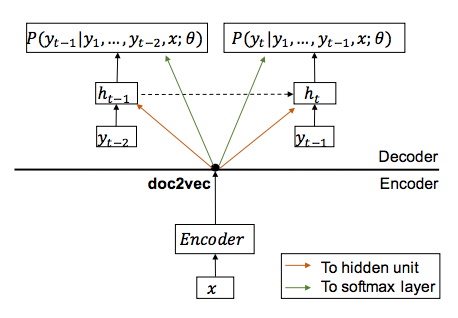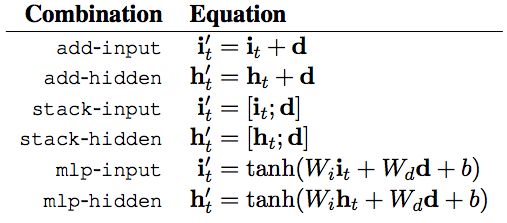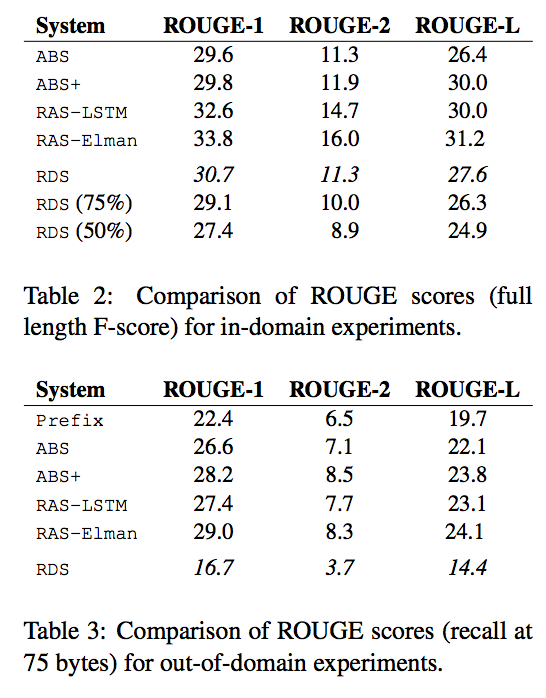#### Paper-pdf

Authors: Xu, et al (Monash, IBM Research, Univ. of Melbourne), Multiling 2017

#### Hypothesis

• Decoupling encoder and decoder can significantly reduce the parameters of the model while yielding competitive results.

#### Background

Let $\mathbf{x}$ be the input and $\mathbf{y}=\{y_1,…,y_n\}$ a sequence of words of length $N$. At decoding time:

\begin{align} \mathbf{y} = P(\mathbf{y} | \mathbf{x};\theta) \end{align}

\begin{align} P(\mathbf{y} | \mathbf{x};\theta)=\prod_{i=1}^N P(y_t | \{y_1,…,y_{t-1}\},\mathbf{x};\theta) \end{align}

For the recurrent decoder:

\begin{align} P(y_t | \{y_1,…,y_{t-1}\},\mathbf{x};\theta)=g_\theta(\mathbf{h}_t, \mathbf{c}_t) \end{align}

$h_t=g_\theta(y_{t-1}, \mathbf{h}_{t-1}, c_t)$: hidden state of the rnn, $c_t$: output of the encoder at time $t$ (context vector)

Attentive encoder: $c_t$ is computed by attending to some of the source words using the previous hidden state $h_{t-1}$.

#### Decoupled encoder-decoder

Encoding and decoding are used in a pipeline. Doc2Vec is used to encode the input (the methods is somehow similar to Rush’s bag of words encoder, instead here the authors use Doc2Vec).Decoder is an LSTM. They combine the input and/or hidden state of the LSTM with the input encoded by Doc2Vec.

They use several strategies for combining (addition, stacking and MLP).#### Results

The results are close to the state-of-the-art for in-domain (Gigaword) experiments. However, for out-of-domain (DUC) they perform poor. RDS is their method, RDS (75\%) uses 75\% of the data.#### Recap

The encoder is essentially very similar to that of Rush’s work (using only n-grams). However, here an unsupervised representation learning method (doc2vec) is used instead of a coupled encoder. Training efficiency was improved from 4 days to 2 days (with the 75% model).